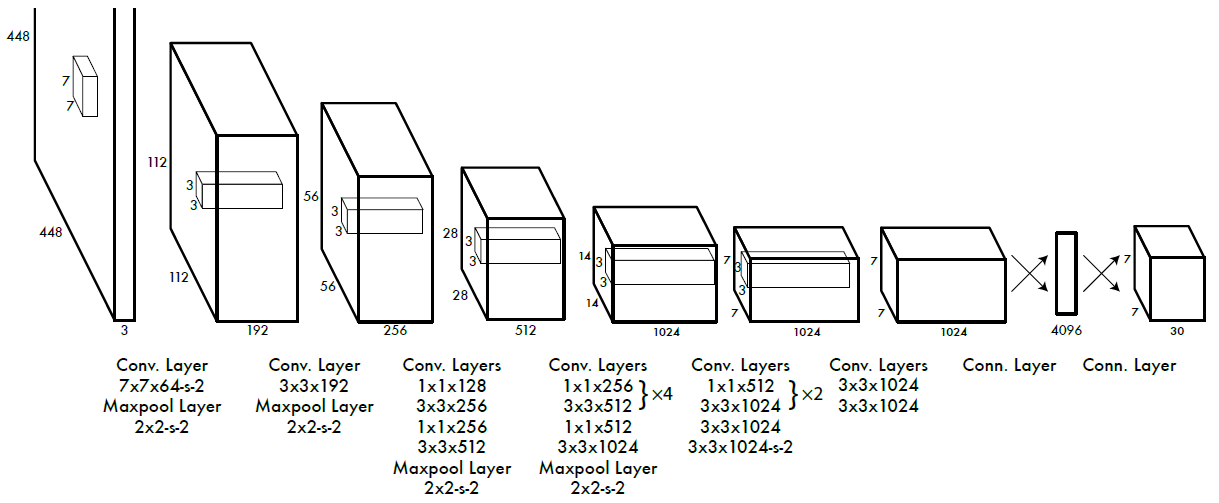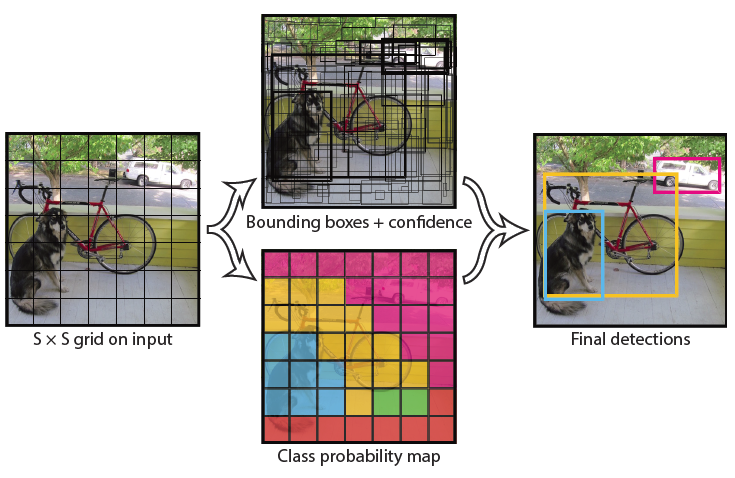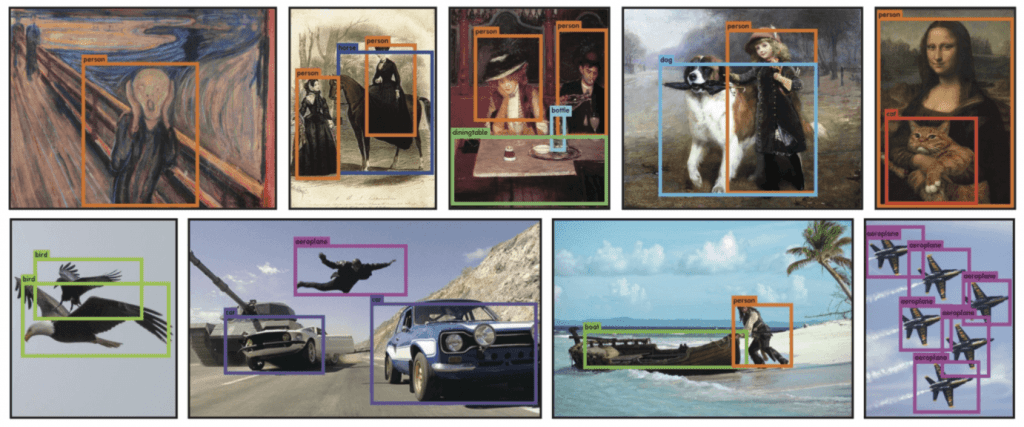# YOLOX Explanation — What is YOLO and What Makes It Special?

This article is the first in the series where I thoroughly explain how the YOLOX (You Only Look Once X) model works. I also coded the model from scratch. If you are interested in the code, you can find a link to it below:

This series has 4 parts to fully go over the YOLOX algorithm:

To completely understand the entire series, you will need to know:

# What Does The YOLO Algorithm Solve?

To start, let’s go over the issue YOLO attempts to solve.

The YOLO algorithm was first introduced in 2015 to solve the object detection problem. As the name suggests, the object detection problem is when a computer is given an image and has to detect where certain objects in that image are.

To solve the object detection problem, YOLO uses bounding boxes. A bounding box is a box put around a part of an image to show that there is an object in the boxed part of the image. For example, imagine I wanted to know where my cat was in this picture:

The bounding box enclosing my cat may look like the following:

What if instead, I wanted to locate my cat’s ears and eyes? Then there might be 4 boxes that look like the following:

This task is easy enough for humans, but for a computer, this task is not very intuitive. Some of the issues that a computer faces are:

• Not having a set number of bounding boxes to put on an image
• Having to put different size bounding boxes on an image
• Needed to understand what an object looks like at different scales

# How Does YOLO Work?

The YOLO algorithm works by predicting three different features:

1. The location of the bounding box on the image
2. The confidence that there’s an object in the bounding box (Note: this is the confidence for any object the model knows about to be in the box, not for a specific class to be in the box)
3. The class or label of the object in the box

So, the bounding box around my cat’s face may have the following properties:

1. Bounding box: (25, 5, 185, 183)
2. Confidence: 0.9567
3. Class: cat

Notice how the bounding box is broken up into 4 parts. In some bounding box algorithms, the bounding box has the following properties:

(x coordinate of top left corner, y coordinate of top left corner, width of bounding box, height of bounding box)

Other bounding box algorithms may use the following properties:

(x coordinate of top left corner, y coordinate of top left corner, x coordinate of bottom right corner, y coordinate of bottom right corner)

Either way, the predictions essentially mean the same thing.

# How Does YOLO Make Predictions?

The original YOLO algorithm had the goal of processing an image quickly and making accurate predictions. The speed is necessary for real-time tasks such as self-driving vehicles which need to know where an object is immediately.

The YOLO algorithm treats the bounding box problem as a regression task and a classification task which takes the original image as input and outputs the 3 predictions listed above.

In this article, I am just going to briefly explain how YOLOv1 does this task. In the next article, I will go more in-depth as to how an improved version of this algorithm compares to YOLOX.

The YOLOv1 algorithm takes the image as input and outputs a tensor that can be broken up into the three properties of a bounding box: location, confidence, and class:Fig 1. Picture from the original paper: https://paperswithcode.com/method/yolov1

Notice how the input is a 448×448×3 tensor. This tensor is essentially an image with a 448 pixel and 448 pixel height. The 3 comes from the RGB values. So, a colored image can be broken up into 3 tensors where each of these three tensors represents the R, G, and B values in the image.

The output is a 7×7×30 tensor. Before going into what this tensor represents, let’s see how the authors define the bounding boxes:Fig 2. Picture from the original paper: https://paperswithcode.com/method/yolov1

The authors of the YOLO algorithm use a grid of S×S where S is 7. Notice that the output of the network shown in Fig 1. is also S×S or 7×7. The output of the network is the 7×7 grid where each part of the 7×7 grid is a different bounding box prediction totaling 49 bounding box predictions.

Each part of the 7×7 grid has 30 values. The authors state that “[the model] divides the image into an S×S grid and for each grid cell predicts B bounding boxes, confidence for those boxes, and C class probabilities. These predictions are encoded as an S × S × (B ∗ 5 + C) tensor.”

So, each 30 element tensor can be broken up into classes C, and bounding boxes B. In the paper, the authors use B=2 and C=20 (which is where 30 comes from: B*5 + C = 2*5 + 20 = 10 + 20 = 30).

The C part of the tensor is the probability that the class in the bounding box is the ith class. Since the C part has 20 elements, the model is predicting that 1 of 20 classes is in the bounding box. To get a class prediction, one can take the index of the highest value in the vector and use that index as the class. The index 1–20 can be mapped from numbers to actual classes. For example, 1 may be a dog and 15 may be a cat.

The B part of the tensor is split up into 5 values:

1. The confidence — how confident is that model that there’s an object in the box?
2. x — The x-axis location of the center of the bounding box
3. y — The y-axis location of the center of the bounding box
4. w — The width of the bounding box
5. h — The height of the bounding box

When given an image, the YOLO algorithm removes bounding boxes it is not confident in and keeps the bounding boxes that it is confident in. (of course this is an over simplifaction to avoid this article being too long since the goal is to explain YOLOX, not YOLOv1) Below are some results from the YOLOv1 model:Picture from the original paper: https://paperswithcode.com/method/yolov1

# What Makes YOLO Special?

When YOLO was first released, it was a revolutionary algorithm that had better speed than the SOTA (State Of The Art) models, but also had better accuracy as it made minimal background prediction errors.

Future YOLO algorithms improved the accuracy and/or the speed of the model making it one of the best object detection algorithms and is why I want to talk about one of the newest additions to the series: YOLOX.

In the next article, I will explain exactly how the YOLOX model works.

--

--